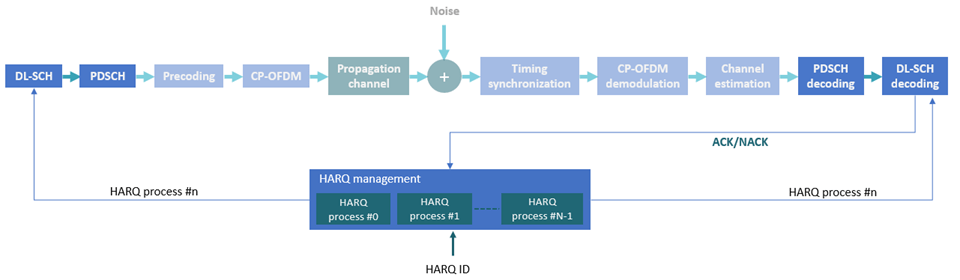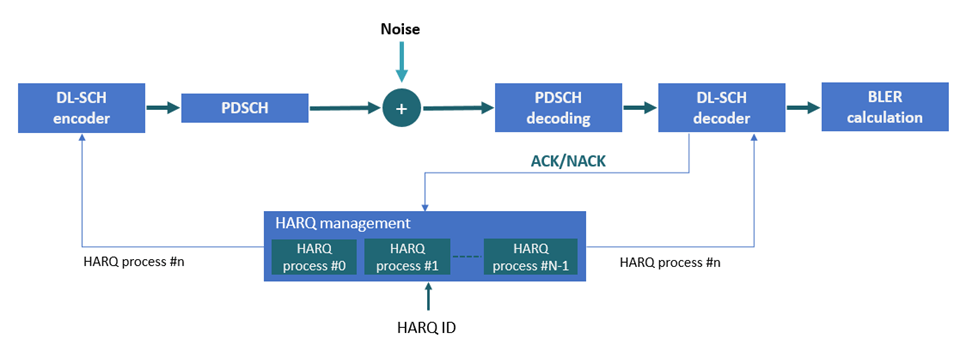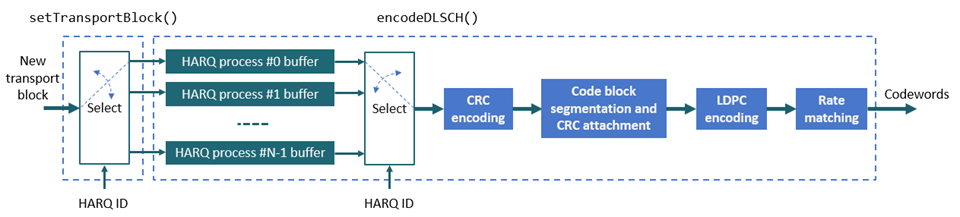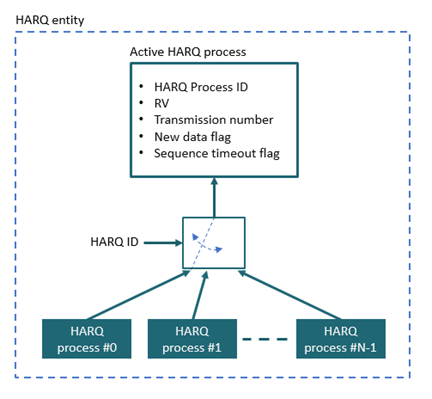# Model 5G NR Transport Channels with HARQ

This example shows how to model 5G NR transport channels with multiple hybrid automatic repeat-request (HARQ) processes using the downlink shared channel (DL-SCH) encoder and decoder 5G Toolbox™ System objects.

### Introduction

This figure shows the link elements that are modeled in this example in the context of a 5G downlink link. These elements are:

• DL-SCH encoding and decoding

• Physical downlink shared channel (PDSCH) encoding and decoding

• HARQ management

The other link elements are not modeled in this example.The examples also measures the block error rate (BLER) using an AWGN channel. This figure shows all of the link elements modeled in this example followed by the BLER calculation.This figure shows that the DL-SCH encoder uses internal buffers to store the transport blocks for each HARQ process and then selects the active HARQ process buffer content for the encoding. The DL-SCH decoder uses a similar buffering mechanism to store and select HARQ processes.The DL-SCH encoder and decoder do not manage the HARQ processes internally. The example uses the HARQ entity object, `HARQEntity.m`, for HARQ process management. This figure shows the structure of the HARQ entity object### Simulation Parameters

Specify the number of transport blocks to simulate and the signal to noise ratio (SNR).

```noTransportBlocks = 100; SNRdB = 7; % SNR in dB```

Reset random number generator for reproducibility.

`rng("default");`

### DL-SCH Configuration

Specify the code rate, the number of HARQ processes, and the redundancy values (RVs) sequence. This sequence controls the redundancy version retransmissions in case of error.

```% DL-SCH parameters codeRate = 490/1024; NHARQProcesses = 16; % Number of parallel HARQ processes to use rvSeq = [0 2 3 1];```

Create the DL-SCH encoder and decoder objects. To use multiple processes, set the `MultipleHARQProcesses` property to `true` for both objects. To enable retransmissions for multiple HARQ processes, the encoder buffers the input bits. The decoder needs a similar mechanism to enable soft combining of retransmissions for each HARQ process.

```% Create DL-SCH encoder object encodeDLSCH = nrDLSCH; encodeDLSCH.MultipleHARQProcesses = true; encodeDLSCH.TargetCodeRate = codeRate; % Create DL-SCH decoder object decodeDLSCH = nrDLSCHDecoder; decodeDLSCH.MultipleHARQProcesses = true; decodeDLSCH.TargetCodeRate = codeRate; decodeDLSCH.LDPCDecodingAlgorithm = "Normalized min-sum"; decodeDLSCH.MaximumLDPCIterationCount = 6;```

The DL-SCH encoder and decoder objects can model up to 16 HARQ processes. The encoder and decoder objects use the `HARQprocessID` property of the HARQ entity object to identify the active HARQ process when performing any of these operations.

• Setting new transport block to transmit

• Encoding data

• Resetting soft buffers

• Decoding data

### Carrier and PDSCH Configuration

Specify the carrier and PDSCH parameters. These parameters are used for PDSCH encoding and decoding and for calculating the transport block size.

Create a carrier object, specifying the subcarrier spacing (SCS) and the bandwidth (BW).

```% Numerology SCS = 15; % SCS: 15, 30, 60, 120 or 240 (kHz) NRB = 52; % BW in number of RBs (52 RBs at 15 kHz SCS for 10 MHz BW) carrier = nrCarrierConfig; carrier.NSizeGrid = NRB; carrier.SubcarrierSpacing = SCS; carrier.CyclicPrefix = "Normal"; % "Normal" or "Extended"```

Create a PDSCH configuration object. The PDSCH parameters determine the available bit capacity and the transport block size.

```modulation = "16QAM"; % Modulation scheme pdsch = nrPDSCHConfig; pdsch.Modulation = modulation; pdsch.PRBSet = 0:NRB-1; % Assume full band allocation pdsch.NumLayers = 1; % Assume only one layer and one codeword```

### HARQ Management

Create a HARQ entity object to manage the HARQ processes. For each HARQ processes, the object stores these elements:

• HARQ ID number.

• RV.

• Transmission number, which indicates how many times a certain transport block has been transmitted.

• Flag to indicate whether new data is required. New data is required when a transport block is received successfully or if a sequence timeout has occurred (all RV transmissions have failed).

• Flag to indicate whether a sequence timeout has occurred (all RV transmissions have failed).

`harqEntity = HARQEntity(0:NHARQProcesses-1,rvSeq,pdsch.NumCodewords);`

The HARQ entity is used to manage the buffers in the DL-SCH encoder and decoder.

### BER Simulation

Loop over a number of transport blocks. For each transport block:

• Calculate the transport block size in number of bits.

• Generate new data block or reset buffers in the decoder.

• Apply DL-SCH encoding.

• Modulate bits to symbols.

• Apply AWGN.

• Demodulate soft bits (symbols to soft bits).

• Decode the DL-SCH.

• Update the HARQ processes.

```% Initialize loop variables noiseVar = 1./(10.^(SNRdB/10)); % Noise variance numBlkErr = 0; % Number of block errors numRxBits = []; % Number of successfully received bits per transmission txedTrBlkSizes = []; % Number of transmitted info bits per transmission for nTrBlk = 1:noTransportBlocks % A transport block or transmission time interval (TTI) corresponds to % one slot carrier.NSlot = carrier.NSlot+1;```

#### Transport Block Size Calculation

Calculate the transport block size.

``` % Generate PDSCH indices info, which is used to calculate the transport % block size [~,pdschInfo] = nrPDSCHIndices(carrier,pdsch); % Calculate transport block sizes Xoh_PDSCH = 0; trBlkSizes = nrTBS(pdsch.Modulation,pdsch.NumLayers,numel(pdsch.PRBSet),pdschInfo.NREPerPRB,codeRate,Xoh_PDSCH);```

Because the PDSCH capacity in bits, `pdsch.G`, is dynamically determined, the actual code rate might not be exactly equal to the target code rate specified by the `TargetCodeRate` property of the `encodeDLSCH` object.

#### HARQ Processing (Buffer Management)

This section explains the buffer management in the encoder and decoder.

• DL-SCH encoder buffers: Generate a new transport block if new data is required for the active HARQ process. Store the transport block in the corresponding buffer. If no new data is required, the buffered bits in the DL-SCH encoder are used for retransmission.

• DL-SCH decoder buffers: The soft buffers in the receiver store previously received versions of the same transport block. These buffers are cleared automatically upon successful reception (no CRC error). However, if the RV sequence ends without successful decoding, the buffers must be flushed manually by calling the `resetSoftBuffer` object function.

``` % Get new transport blocks and flush decoder soft buffer, as required for cwIdx = 1:pdsch.NumCodewords if harqEntity.NewData(cwIdx) % Create and store a new transport block for transmission trBlk = randi([0 1],trBlkSizes(cwIdx),1); setTransportBlock(encodeDLSCH,trBlk,cwIdx-1,harqEntity.HARQProcessID); % If the previous RV sequence ends without successful decoding, % flush the soft buffer explicitly if harqEntity.SequenceTimeout(cwIdx) resetSoftBuffer(decodeDLSCH,cwIdx-1,harqEntity.HARQProcessID); end end end```

#### DL-SCH Encoding

Encode the DL-SCH transport blocks.

``` codedTrBlock = encodeDLSCH(pdsch.Modulation,pdsch.NumLayers,pdschInfo.G, ... harqEntity.RedundancyVersion,harqEntity.HARQProcessID);```

#### PDSCH Encoding

Generate the PDSCH symbols.

` modOut = nrPDSCH(carrier,pdsch,codedTrBlock);`

#### AWGN Channel

` rxSig = awgn(modOut,SNRdB); `

#### PDSCH Demodulation

` rxLLR = nrPDSCHDecode(carrier,pdsch,rxSig,noiseVar);`

#### DL-SCH Decoding

Apply DL-SCH decoding.

``` decodeDLSCH.TransportBlockLength = trBlkSizes; [decbits,blkerr] = decodeDLSCH(rxLLR,pdsch.Modulation,pdsch.NumLayers, ... harqEntity.RedundancyVersion,harqEntity.HARQProcessID);```

#### Results

Store the results to calculate the BLER.

``` % Store values to calculate throughput (only for active transport blocks) if(any(trBlkSizes ~= 0)) numRxBits = [numRxBits trBlkSizes.*(1-blkerr)]; txedTrBlkSizes = [txedTrBlkSizes trBlkSizes]; end if blkerr numBlkErr = numBlkErr + 1; end```

#### HARQ Process Update

Update the current HARQ process with the CRC error, and then advance to the next process. This step updates the information related to the active HARQ process in the HARQ entity.

` statusReport = updateAndAdvance(harqEntity,blkerr,trBlkSizes,pdschInfo.G); `

Display information about the current decoding attempt.

``` disp("Slot "+(nTrBlk)+". "+statusReport); end % for nTrBlk = 1:noTransportBlocks```
```Slot 1. HARQ Proc 0: CW0: Initial transmission failed (RV=0,CR=0.481509). Slot 2. HARQ Proc 1: CW0: Initial transmission failed (RV=0,CR=0.481509). Slot 3. HARQ Proc 2: CW0: Initial transmission failed (RV=0,CR=0.481509). Slot 4. HARQ Proc 3: CW0: Initial transmission failed (RV=0,CR=0.481509). Slot 5. HARQ Proc 4: CW0: Initial transmission failed (RV=0,CR=0.481509). Slot 6. HARQ Proc 5: CW0: Initial transmission failed (RV=0,CR=0.481509). Slot 7. HARQ Proc 6: CW0: Initial transmission failed (RV=0,CR=0.481509). Slot 8. HARQ Proc 7: CW0: Initial transmission failed (RV=0,CR=0.481509). Slot 9. HARQ Proc 8: CW0: Initial transmission passed (RV=0,CR=0.481509). Slot 10. HARQ Proc 9: CW0: Initial transmission passed (RV=0,CR=0.481509). Slot 11. HARQ Proc 10: CW0: Initial transmission failed (RV=0,CR=0.481509). Slot 12. HARQ Proc 11: CW0: Initial transmission passed (RV=0,CR=0.481509). Slot 13. HARQ Proc 12: CW0: Initial transmission failed (RV=0,CR=0.481509). Slot 14. HARQ Proc 13: CW0: Initial transmission failed (RV=0,CR=0.481509). Slot 15. HARQ Proc 14: CW0: Initial transmission failed (RV=0,CR=0.481509). Slot 16. HARQ Proc 15: CW0: Initial transmission failed (RV=0,CR=0.481509). Slot 17. HARQ Proc 0: CW0: Retransmission #1 passed (RV=2,CR=0.481509). Slot 18. HARQ Proc 1: CW0: Retransmission #1 passed (RV=2,CR=0.481509). Slot 19. HARQ Proc 2: CW0: Retransmission #1 passed (RV=2,CR=0.481509). Slot 20. HARQ Proc 3: CW0: Retransmission #1 passed (RV=2,CR=0.481509). Slot 21. HARQ Proc 4: CW0: Retransmission #1 passed (RV=2,CR=0.481509). Slot 22. HARQ Proc 5: CW0: Retransmission #1 passed (RV=2,CR=0.481509). Slot 23. HARQ Proc 6: CW0: Retransmission #1 passed (RV=2,CR=0.481509). Slot 24. HARQ Proc 7: CW0: Retransmission #1 passed (RV=2,CR=0.481509). Slot 25. HARQ Proc 8: CW0: Initial transmission passed (RV=0,CR=0.481509). Slot 26. HARQ Proc 9: CW0: Initial transmission passed (RV=0,CR=0.481509). Slot 27. HARQ Proc 10: CW0: Retransmission #1 passed (RV=2,CR=0.481509). Slot 28. HARQ Proc 11: CW0: Initial transmission passed (RV=0,CR=0.481509). Slot 29. HARQ Proc 12: CW0: Retransmission #1 passed (RV=2,CR=0.481509). Slot 30. HARQ Proc 13: CW0: Retransmission #1 passed (RV=2,CR=0.481509). Slot 31. HARQ Proc 14: CW0: Retransmission #1 passed (RV=2,CR=0.481509). Slot 32. HARQ Proc 15: CW0: Retransmission #1 passed (RV=2,CR=0.481509). Slot 33. HARQ Proc 0: CW0: Initial transmission failed (RV=0,CR=0.481509). Slot 34. HARQ Proc 1: CW0: Initial transmission failed (RV=0,CR=0.481509). Slot 35. HARQ Proc 2: CW0: Initial transmission failed (RV=0,CR=0.481509). Slot 36. HARQ Proc 3: CW0: Initial transmission failed (RV=0,CR=0.481509). Slot 37. HARQ Proc 4: CW0: Initial transmission passed (RV=0,CR=0.481509). Slot 38. HARQ Proc 5: CW0: Initial transmission failed (RV=0,CR=0.481509). Slot 39. HARQ Proc 6: CW0: Initial transmission failed (RV=0,CR=0.481509). Slot 40. HARQ Proc 7: CW0: Initial transmission failed (RV=0,CR=0.481509). Slot 41. HARQ Proc 8: CW0: Initial transmission failed (RV=0,CR=0.481509). Slot 42. HARQ Proc 9: CW0: Initial transmission passed (RV=0,CR=0.481509). Slot 43. HARQ Proc 10: CW0: Initial transmission failed (RV=0,CR=0.481509). Slot 44. HARQ Proc 11: CW0: Initial transmission passed (RV=0,CR=0.481509). Slot 45. HARQ Proc 12: CW0: Initial transmission failed (RV=0,CR=0.481509). Slot 46. HARQ Proc 13: CW0: Initial transmission passed (RV=0,CR=0.481509). Slot 47. HARQ Proc 14: CW0: Initial transmission failed (RV=0,CR=0.481509). Slot 48. HARQ Proc 15: CW0: Initial transmission failed (RV=0,CR=0.481509). Slot 49. HARQ Proc 0: CW0: Retransmission #1 passed (RV=2,CR=0.481509). Slot 50. HARQ Proc 1: CW0: Retransmission #1 passed (RV=2,CR=0.481509). Slot 51. HARQ Proc 2: CW0: Retransmission #1 passed (RV=2,CR=0.481509). Slot 52. HARQ Proc 3: CW0: Retransmission #1 passed (RV=2,CR=0.481509). Slot 53. HARQ Proc 4: CW0: Initial transmission failed (RV=0,CR=0.481509). Slot 54. HARQ Proc 5: CW0: Retransmission #1 passed (RV=2,CR=0.481509). Slot 55. HARQ Proc 6: CW0: Retransmission #1 passed (RV=2,CR=0.481509). Slot 56. HARQ Proc 7: CW0: Retransmission #1 passed (RV=2,CR=0.481509). Slot 57. HARQ Proc 8: CW0: Retransmission #1 passed (RV=2,CR=0.481509). Slot 58. HARQ Proc 9: CW0: Initial transmission passed (RV=0,CR=0.481509). Slot 59. HARQ Proc 10: CW0: Retransmission #1 passed (RV=2,CR=0.481509). Slot 60. HARQ Proc 11: CW0: Initial transmission passed (RV=0,CR=0.481509). Slot 61. HARQ Proc 12: CW0: Retransmission #1 passed (RV=2,CR=0.481509). Slot 62. HARQ Proc 13: CW0: Initial transmission failed (RV=0,CR=0.481509). Slot 63. HARQ Proc 14: CW0: Retransmission #1 passed (RV=2,CR=0.481509). Slot 64. HARQ Proc 15: CW0: Retransmission #1 passed (RV=2,CR=0.481509). Slot 65. HARQ Proc 0: CW0: Initial transmission failed (RV=0,CR=0.481509). Slot 66. HARQ Proc 1: CW0: Initial transmission passed (RV=0,CR=0.481509). Slot 67. HARQ Proc 2: CW0: Initial transmission failed (RV=0,CR=0.481509). Slot 68. HARQ Proc 3: CW0: Initial transmission failed (RV=0,CR=0.481509). Slot 69. HARQ Proc 4: CW0: Retransmission #1 passed (RV=2,CR=0.481509). Slot 70. HARQ Proc 5: CW0: Initial transmission failed (RV=0,CR=0.481509). Slot 71. HARQ Proc 6: CW0: Initial transmission failed (RV=0,CR=0.481509). Slot 72. HARQ Proc 7: CW0: Initial transmission failed (RV=0,CR=0.481509). Slot 73. HARQ Proc 8: CW0: Initial transmission passed (RV=0,CR=0.481509). Slot 74. HARQ Proc 9: CW0: Initial transmission passed (RV=0,CR=0.481509). Slot 75. HARQ Proc 10: CW0: Initial transmission failed (RV=0,CR=0.481509). Slot 76. HARQ Proc 11: CW0: Initial transmission passed (RV=0,CR=0.481509). Slot 77. HARQ Proc 12: CW0: Initial transmission failed (RV=0,CR=0.481509). Slot 78. HARQ Proc 13: CW0: Retransmission #1 passed (RV=2,CR=0.481509). Slot 79. HARQ Proc 14: CW0: Initial transmission failed (RV=0,CR=0.481509). Slot 80. HARQ Proc 15: CW0: Initial transmission failed (RV=0,CR=0.481509). Slot 81. HARQ Proc 0: CW0: Retransmission #1 passed (RV=2,CR=0.481509). Slot 82. HARQ Proc 1: CW0: Initial transmission passed (RV=0,CR=0.481509). Slot 83. HARQ Proc 2: CW0: Retransmission #1 passed (RV=2,CR=0.481509). Slot 84. HARQ Proc 3: CW0: Retransmission #1 passed (RV=2,CR=0.481509). Slot 85. HARQ Proc 4: CW0: Initial transmission failed (RV=0,CR=0.481509). Slot 86. HARQ Proc 5: CW0: Retransmission #1 passed (RV=2,CR=0.481509). Slot 87. HARQ Proc 6: CW0: Retransmission #1 passed (RV=2,CR=0.481509). Slot 88. HARQ Proc 7: CW0: Retransmission #1 passed (RV=2,CR=0.481509). Slot 89. HARQ Proc 8: CW0: Initial transmission failed (RV=0,CR=0.481509). Slot 90. HARQ Proc 9: CW0: Initial transmission passed (RV=0,CR=0.481509). Slot 91. HARQ Proc 10: CW0: Retransmission #1 passed (RV=2,CR=0.481509). Slot 92. HARQ Proc 11: CW0: Initial transmission failed (RV=0,CR=0.481509). Slot 93. HARQ Proc 12: CW0: Retransmission #1 passed (RV=2,CR=0.481509). Slot 94. HARQ Proc 13: CW0: Initial transmission passed (RV=0,CR=0.481509). Slot 95. HARQ Proc 14: CW0: Retransmission #1 passed (RV=2,CR=0.481509). Slot 96. HARQ Proc 15: CW0: Retransmission #1 passed (RV=2,CR=0.481509). Slot 97. HARQ Proc 0: CW0: Initial transmission failed (RV=0,CR=0.481509). Slot 98. HARQ Proc 1: CW0: Initial transmission failed (RV=0,CR=0.481509). Slot 99. HARQ Proc 2: CW0: Initial transmission passed (RV=0,CR=0.481509). Slot 100. HARQ Proc 3: CW0: Initial transmission failed (RV=0,CR=0.481509). ```

### BLER Results

Calculate the BLER and the throughput (percentage of successfully received transport blocks). To provide statistically meaningful results, run this simulation for many transport blocks.

```maxThroughput = sum(txedTrBlkSizes); % Maximum possible throughput totalNumRxBits = sum(numRxBits,2); % Number of successfully received bits disp("Block Error Rate: "+string(numBlkErr/noTransportBlocks))```
```Block Error Rate: 0.43 ```
`disp("Throughput: " + string(totalNumRxBits*100/maxThroughput) + "%")`
```Throughput: 57% ```